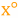# Degree

 Block Group: Trigonometric Functions Icon:The Degree block converts radians to degrees.

For information on using dataflow blocks, see Dataflow.

## Input/Output Property

The following property of the Degree block can take input and give output.

• input (number)

input specifies the angle in radians, to convert to degrees.

## Output Property

The following property of the Degree block can give output but cannot take input.

• output (number)

output returns the input value, converted from radians to degrees.

## Example

The following image shows an example of the Degree block. In this example, pi is converted from radians to degrees.